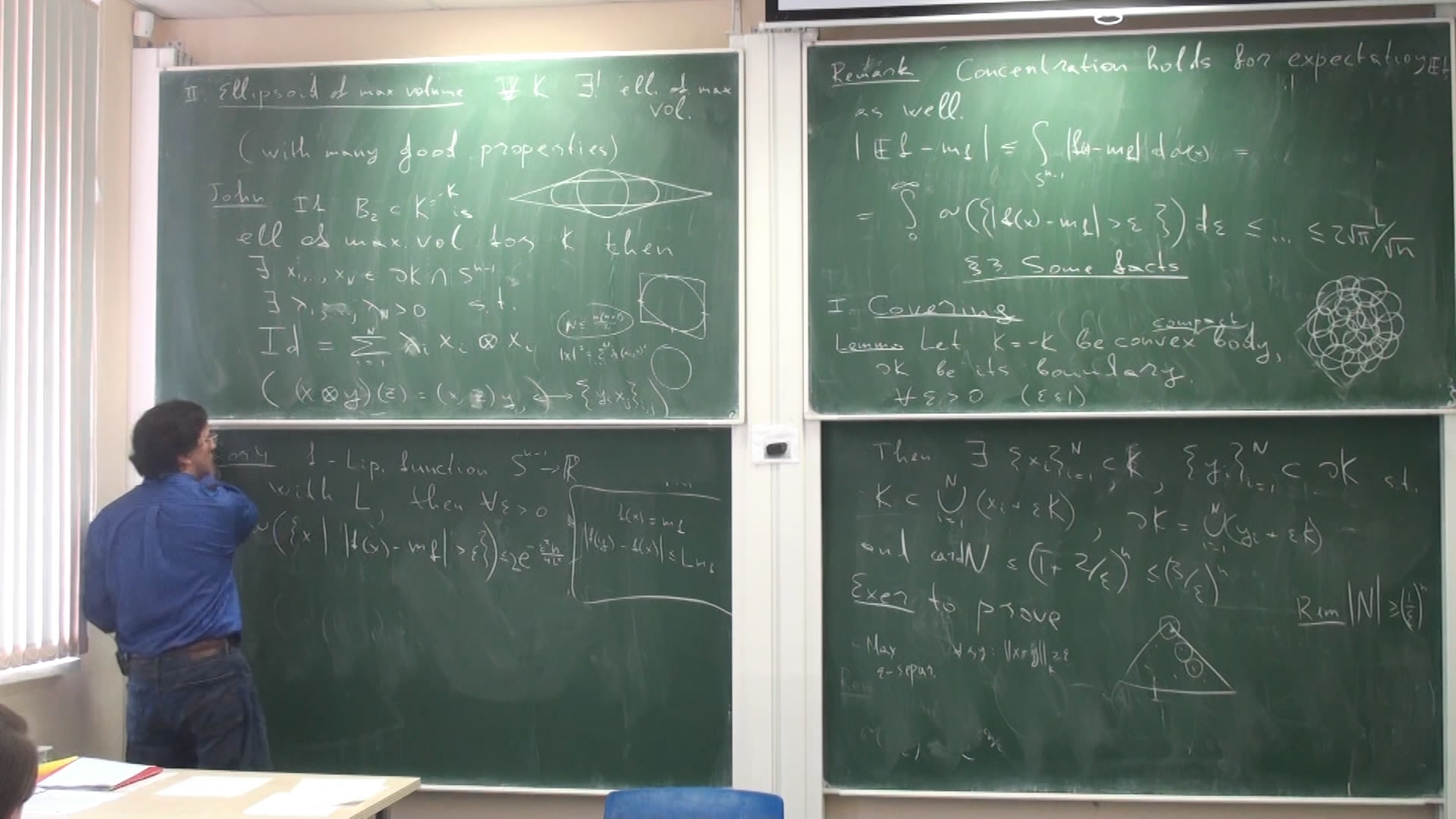# Asymptotic Geometric Analysis (AGA)

Курс
Предмет:The purpose of this mini-course is to provide a brief introduction to some classical results and basic ideas of Asymptotic Geometric Analysis (AGA). AGA  studies asymptotic behaviour of various quantitative parameters of high dimensional
convex bodies, their sections and projections. AGA combines technique from Functional Analysis, Convex Geometry, Combinatorics and Probability. One of the most important results is Dvoretzky's theorem, which states that every N-dimensional convex body has a (ln N)-dimensional section which is almost a Euclidean ball. Milman's proof of the Dvoretzky theorem is based on so-called concentration phenomena, which lies behind many results of the theory. Roughly speaking concentration phenomena says that a Lipschitz function on a "good" measure space (e.g., on the Euclidean sphere endowed with normalized Lebesgue measure) is almost constant in a sense that it can deviate from its average only on set of a very small measure. In our course we discuss concentration phenomenon.

Аннотация курса

2